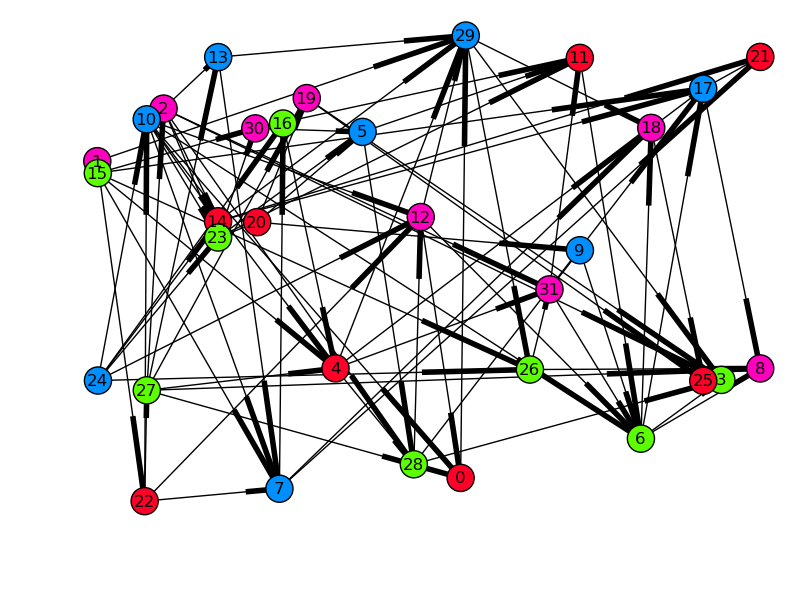#### Previous topic

Parallel examples

#### Next topic

Details of Parallel Computing with IPython

Warning

This documentation is for an old version of IPython. You can find docs for newer versions here.

# DAG Dependencies¶

Often, parallel workflow is described in terms of a Directed Acyclic Graph or DAG. A popular library for working with Graphs is NetworkX. Here, we will walk through a demo mapping a nx DAG to task dependencies.

The full script that runs this demo can be found in examples/parallel/dagdeps.py.

## Why are DAGs good for task dependencies?¶

The ‘G’ in DAG is ‘Graph’. A Graph is a collection of nodes and edges that connect the nodes. For our purposes, each node would be a task, and each edge would be a dependency. The ‘D’ in DAG stands for ‘Directed’. This means that each edge has a direction associated with it. So we can interpret the edge (a,b) as meaning that b depends on a, whereas the edge (b,a) would mean a depends on b. The ‘A’ is ‘Acyclic’, meaning that there must not be any closed loops in the graph. This is important for dependencies, because if a loop were closed, then a task could ultimately depend on itself, and never be able to run. If your workflow can be described as a DAG, then it is impossible for your dependencies to cause a deadlock.

## A Sample DAG¶

Here, we have a very simple 5-node DAG:

With NetworkX, an arrow is just a fattened bit on the edge. Here, we can see that task 0 depends on nothing, and can run immediately. 1 and 2 depend on 0; 3 depends on 1 and 2; and 4 depends only on 1.

A possible sequence of events for this workflow:

1. Task 0 can run right away
2. 0 finishes, so 1,2 can start
3. 1 finishes, 3 is still waiting on 2, but 4 can start right away
4. 2 finishes, and 3 can finally start

Further, taking failures into account, assuming all dependencies are run with the default success=True,failure=False, the following cases would occur for each node’s failure:

1. fails: all other tasks fail as Impossible
2. 2 can still succeed, but 3,4 are unreachable
3. 3 becomes unreachable, but 4 is unaffected
4. and 4. are terminal, and can have no effect on other nodes

The code to generate the simple DAG:

```import networkx as nx

G = nx.DiGraph()

# add 5 nodes, labeled 0-4:
# 1,2 depend on 0:
# 3 depends on 1,2
# 4 depends on 1

# now draw the graph:
pos = { 0 : (0,0), 1 : (1,1), 2 : (-1,1),
3 : (0,2), 4 : (2,2)}
nx.draw(G, pos, edge_color='r')
```

For demonstration purposes, we have a function that generates a random DAG with a given number of nodes and edges.

```def random_dag(nodes, edges):
"""Generate a random Directed Acyclic Graph (DAG) with a given number of nodes and edges."""
G = nx.DiGraph()
for i in range(nodes):
while edges > 0:
a = randint(0,nodes-1)
b=a
while b==a:
b = randint(0,nodes-1)
if nx.is_directed_acyclic_graph(G):
edges -= 1
else:
# we closed a loop!
G.remove_edge(a,b)
return G
```

So first, we start with a graph of 32 nodes, with 128 edges:

```In : G = random_dag(32,128)
```

Now, we need to build our dict of jobs corresponding to the nodes on the graph:

```In : jobs = {}

# in reality, each job would presumably be different
# randomwait is just a function that sleeps for a random interval
In : for node in G:
...:     jobs[node] = randomwait
```

Once we have a dict of jobs matching the nodes on the graph, we can start submitting jobs, and linking up the dependencies. Since we don’t know a job’s msg_id until it is submitted, which is necessary for building dependencies, it is critical that we don’t submit any jobs before other jobs it may depend on. Fortunately, NetworkX provides a topological_sort() method which ensures exactly this. It presents an iterable, that guarantees that when you arrive at a node, you have already visited all the nodes it on which it depends:

```In : rc = Client()

In : results = {}

In : for node in G.topological_sort():
...:    # get list of AsyncResult objects from nodes
...:    # leading into this one as dependencies
...:    deps = [ results[n] for n in G.predecessors(node) ]
...:    # submit and store AsyncResult object
...:    with view.temp_flags(after=deps, block=False):
...:         results[node] = view.apply_with_flags(jobs[node])
```

Now that we have submitted all the jobs, we can wait for the results:

```In : view.wait(results.values())
```

Now, at least we know that all the jobs ran and did not fail (r.get() would have raised an error if a task failed). But we don’t know that the ordering was properly respected. For this, we can use the metadata attribute of each AsyncResult.

These objects store a variety of metadata about each task, including various timestamps. We can validate that the dependencies were respected by checking that each task was started after all of its predecessors were completed:

```def validate_tree(G, results):
"""Validate that jobs executed after their dependencies."""
for node in G:
for parent in G.predecessors(node):
assert started > finished, "%s should have happened after %s"%(node, parent)
```

We can also validate the graph visually. By drawing the graph with each node’s x-position as its start time, all arrows must be pointing to the right if dependencies were respected. For spreading, the y-position will be the runtime of the task, so long tasks will be at the top, and quick, small tasks will be at the bottom.

```In : from matplotlib.dates import date2num

In : from matplotlib.cm import gist_rainbow

In : pos = {}; colors = {}

In : for node in G: